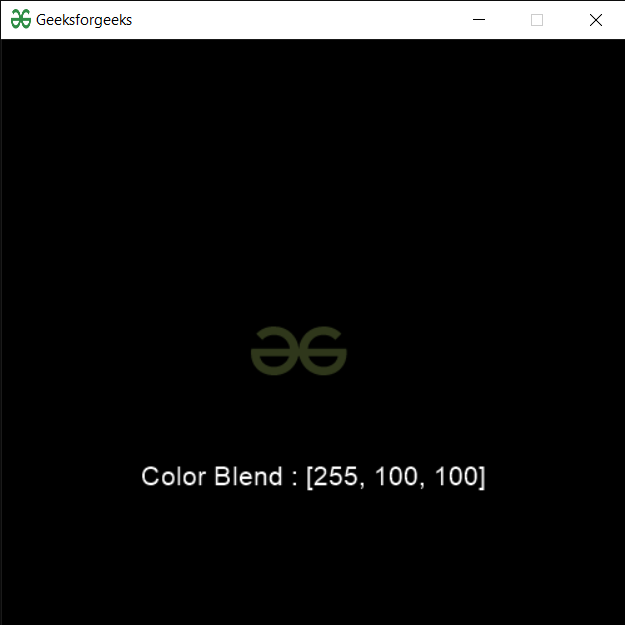Open In App

# PYGLET – Sprite Blend Color

In this article we will see how we can access blend color of the sprite in PYGLET module in python. Pyglet is easy to use but powerful library for developing visually rich GUI applications like games, multimedia etc. A window is a “heavyweight” object occupying operating system resources. Windows may appear as floating regions or can be set to fill an entire screen (fullscreen). A sprite is an instance of an image displayed on-screen. Multiple sprites can display the same image at different positions on the screen. Sprites can also be scaled larger or smaller, rotated at any angle and drawn at a fractional opacity. Image is loaded with the help of image module of pyglet. Blend property sets the color of the sprite’s vertices. This allows the sprite to be drawn with a color tint. The color is specified as an RGB tuple of integers ‘(red, green, blue)’. Each color component must be in the range 0 (dark) to 255 (saturated).
We can create a window and sprite object with the help of commands given below

```# creating a window
window = pyglet.window.Window(width, height, title)

# creating a sprite object
sprite = pyglet.sprite.Sprite(img, x, y)```

In order to create window we use color attribute with sprite object
Syntax : sprite.color
Argument : It takes no argument
Return : It returns tuple

Below is the implementation

## Python3

 `# importing pyglet module``import` `pyglet``import` `pyglet.window.key as key``  ` `# width of window``width ``=` `500``  ` `# height of window``height ``=` `500``  ` `# caption i.e title of the window``title ``=` `"Geeksforgeeks"``  ` `# creating a window``window ``=` `pyglet.window.Window(width, height, title)``  ` `# text ``text ``=` `"Welcome to GeeksforGeeks"`` ` `# creating label with following properties``# font = cooper``# position = 250, 150``# anchor position = center``label ``=` `pyglet.text.Label(text,``                          ``font_name ``=``'Cooper'``,``                          ``font_size ``=` `16``,``                          ``x ``=` `250``, ``                          ``y ``=` `150``,``                          ``anchor_x ``=``'center'``, ``                          ``anchor_y ``=``'center'``)`  `# creating a batch``batch ``=` `pyglet.graphics.Batch()` `# loading geeksforgeeks image``image ``=` `pyglet.image.load(``'gfg.png'``)`   `# creating sprite object``# it is instance of an image displayed on-screen``sprite ``=` `pyglet.sprite.Sprite(image, x ``=` `200``, y ``=` `230``)``  ` `# on draw event``@window``.event``def` `on_draw():``      ` `    ``# clear the window``    ``window.clear()``      ` `    ``# draw the label``    ``label.draw()``    ` `    ``# draw the image on screen``    ``sprite.draw()``      ` `# key press event    ``@window``.event``def` `on_key_press(symbol, modifier):``  ` `    ``# key "C" get press``    ``if` `symbol ``=``=` `key.C:``        ` `        ``# printing the message``        ``print``(``"Key : C is pressed"``)``        ` `# image for icon``img ``=` `image ``=` `pyglet.resource.image(``"gfg.png"``)` `# setting image as icon``window.set_icon(img)` `# accessing color blend property of the sprite``sprite.color ``=` `(``255``, ``100``, ``100``)` `# setting text to the label``text ``=` `"Color Blend : "` `+` `str``(sprite.color)``label.text ``=` `text``   ` `# start running the application``pyglet.app.run()`

Output :